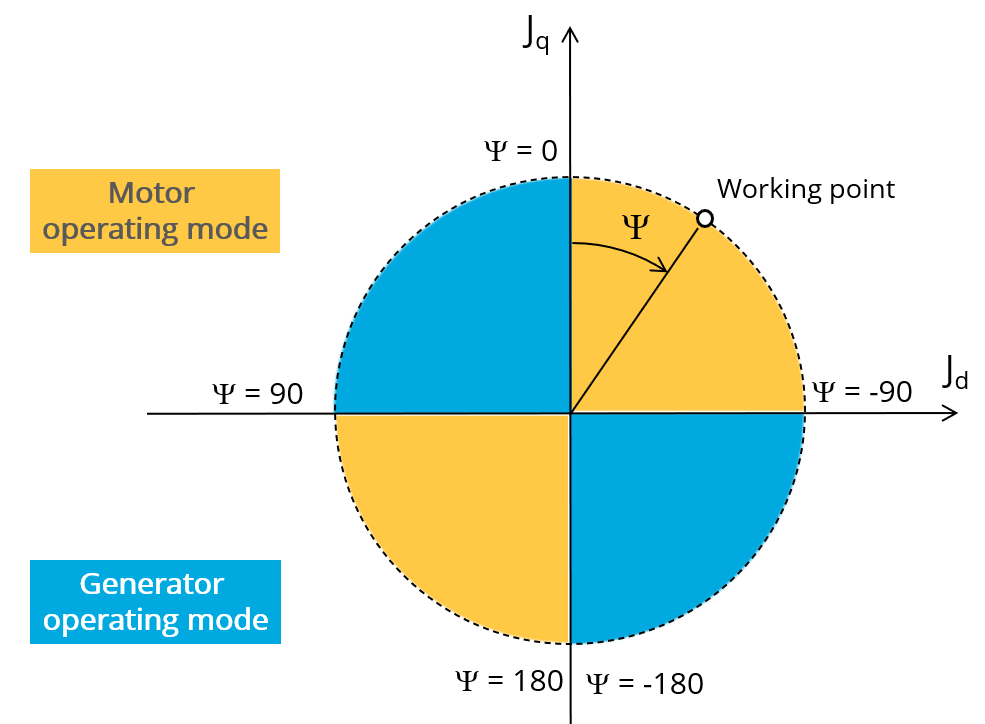# Inputs

## 1. Standard inputs

1. Line current, rms

The line current supplied to the machine: “Line current, rms” ( Line current, rms value ) must be provided.

2. Control angle

Considering the vector diagram shown below, the “ Control angle ” is the angle between the electromotive force E and the electrical current (J) (Ψ = (E, J)).

It is an electrical angle. The default value is 45 degrees. It must be set in a range of -90 to 90 degrees.

This range of values covers all the possible working point in motor convention.Definition of the control angle Ψ - Motor convention
3. Speed

The imposed “ Speed ” ( Speed ) of the machine must be set.

4. Represented coil conductors

In transient application, it is possible to export a project into Flux® environment where the elementary wires will be modeled with solid conductors. The geometry, the meshing and the corresponding electric circuit will be defined to well represent them.

One choices is possible:

• “All phases”: The elementary wires will be represented into all the phases

The list of advanced inputs dedicated to this export are listed below.

For more details, please refer to the section dedicated to the List of generic advanced inputs.

1. Number of computations per electrical period

The default value is equal to 50. The minimum allowed value is 13.

2. Number of computed electrical periods

The default value is equal to 2. The minimum allowed value is 1 and the maximum value is equal to 10.

3. Mesh order

The default level is second order mesh.

4. Airgap mesh coefficient

Airgap mesh coefficient is set to 1.5 by default.

## 3. List of generic advanced inputs

1. Number of computations per electrical period

The number of computations per electrical period “ No. comp. / elec. period ” (Number of computations per electrical period) influences the accuracy of results and the computation time.

The default value is 50. The minimum allowed value is 13. This default value provides a good compromise between the accuracy of results and computation time.

2. Number of computed electrical periods

The default value is 2. The minimum allowed value is 1 and the maximum value is equal to 10.

3. Mesh order

To get results, Finite Element Modelling computations are performed.

The geometry of the machine is meshed.

Two levels of meshing can be considered: First order and second order.

The default level is second order mesh.

4. Airgap mesh coefficient

The advanced user input “ Airgap mesh coefficient ” is a coefficient which adjusts the size of mesh elements inside the airgap. When you decrease the value of “ Airgap mesh coefficient ”, you reduce the size of mesh elements, thus increasing the mesh density inside the airgap and the accuracy of results.

The imposed Mesh Point (size of mesh elements touching points of the geometry) is described with the following parameters:

MeshPoint = (airgap) x (airgap mesh coefficient)

Airgap mesh coefficient is set to 1.5 by default.

The variation range of values for this parameter is [0.05; 2].

0.05 giving a very high mesh density and 2 giving a very coarse mesh density.

CAUTION:
Be aware, a very high mesh density does not always mean a better result quality.

However, this always leads to a huge number of nodes in the corresponding finite element model. So, it means a need of huge numerical memory and increases the computation time considerably.

5. Rotor d-axis location

The computations are performed by considering a relative angular position between rotor and stator.

For the reluctance synchronous machines, the rotor d-axis location is defined and automatically used to perform computations.

This value is characterized by the saliency topology. This is important to keep in mind this information it.

For more details, please refer to the document: MotorFactory_2022.3_SMRSM_IR_3PH_Test_Introduction – section “Rotor and stator relative position”.